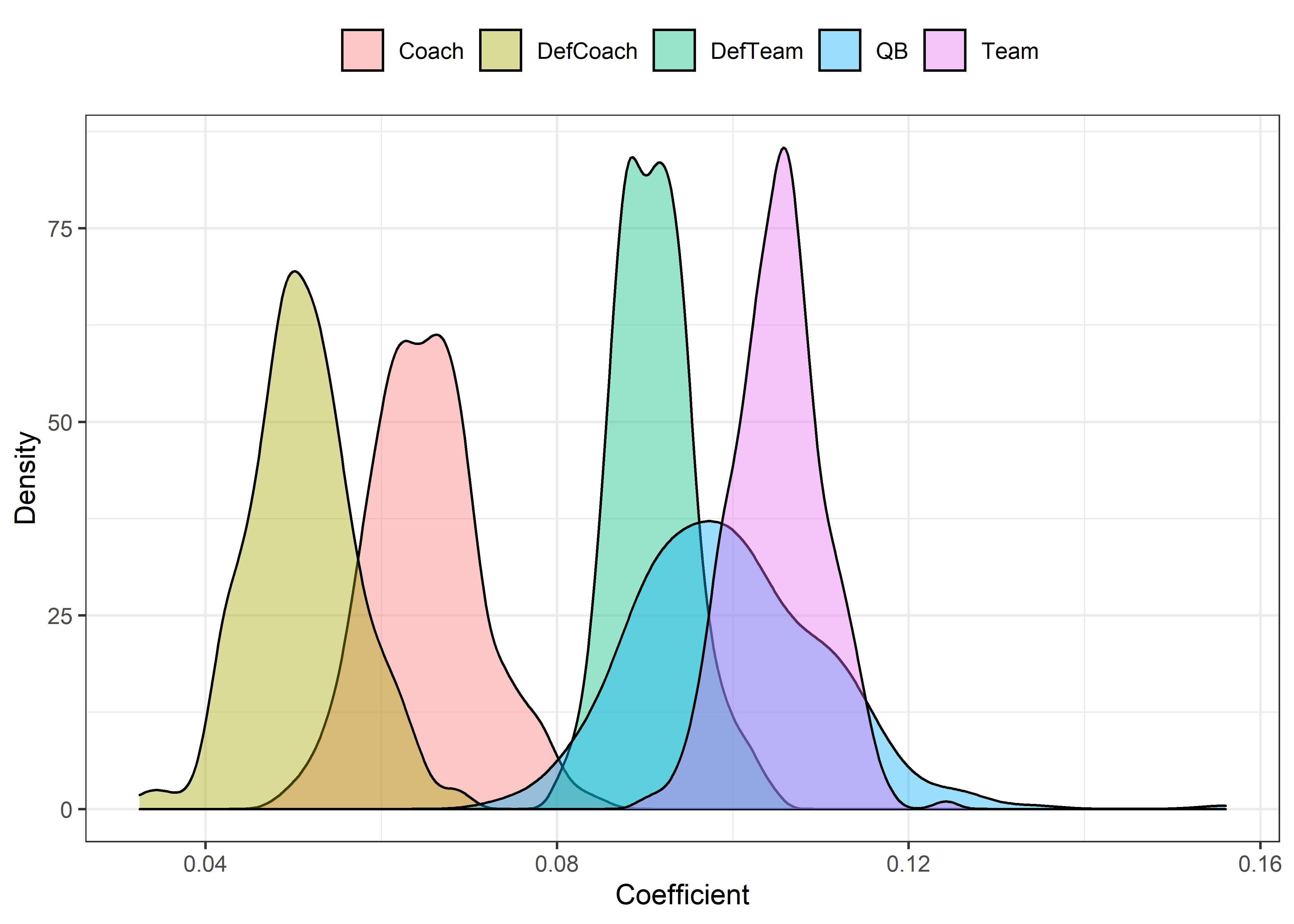-

# Intro

This is a “test” post that I decided to use to show people how I create HTML-format posts using R Markdown. People can follow the source code here.

This is the YAML front matter. Add this to line 1, including the `---` at start and end.

``````---
date: "11-22-2020"

output:
html_document:
toc: yes
toc_depth: '3'
df_print: paged
distill::distill_article:
self_contained: no
toc: yes
toc_depth: 3
---``````

To format R code chunks, use this code add ``` at start and end:

``````{r setup, include=FALSE}
knitr::opts_chunk\$set(
echo = TRUE,
message = FALSE,
warning = FALSE,
dpi = 600,
tidy = 'styler'
)
``````

# Font

This is the code I used to set the font size, right after the front matter.

``````<style type="text/css">
body{
font-size: 14pt;
}
</style>
``````

# Non-R code

To add non-R code without it being run, like I did with front matter and font matter, add ``` at the start and end of such code.

# R code

You can run R code by inserting R code chunks. Refer to my source code.

You can change each block of code’s configurations by clicking on the engine icon on the top-right of the block. Show output only, show code and output, show nothing (run code), show nothing (don’t run code). If you want to show R code without running it, just use the ``` method we did earlier.

R code not to run

``````seasons <- 2000:2019
pbp <- purrr::map_df(seasons, function(x) {
url(
glue::glue("https://raw.githubusercontent.com/guga31bb/nflfastR-data/master/data/play_by_play_{x}.rds")
)
)
})``````

R code to run

``print("This is a code block")``
``##  "This is a code block"``

You can print tables and plots:

Table

``````r <- read.csv("roster_data.csv")
r``````

Plots

``````library(ggplot2)

p <- ggplot(plot, aes(x = coef, fill = effect)) +
geom_density(alpha = .4) +
theme_bw() +
theme(
legend.position = "top",
legend.title = element_blank()
) +
labs(x = "Coefficient", y = "Density") +
ggsave("plot.png", dpi = 600, width = 8, height = 5, units = "in")

p````````# ![External image.](plot.png)``

# Insert images

You can also insert images using the following code. Don’t add it to a code block.

``![External image.](path/image.png)``

Result: